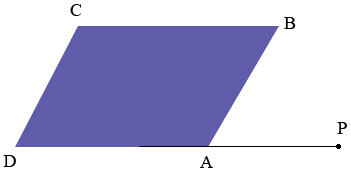SEARCH HOMEMath Central Quandaries & QueriesQuestion from a student: one angle of a parallelogram is four times the measure of the exterior angle adjacent to it. find the interior anglesHi,

An external angle in a polygon is defined in our glossary of mathematical terms. In the diagram below the edge $DA$ of the parallelogram is extended to $P$ to form the exterior angle $BA$ which is adjacent to the interior angle $DAP.$Can you complete the problem now?

PennyMath Central is supported by the University of Regina and The Pacific Institute for the Mathematical Sciences.